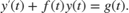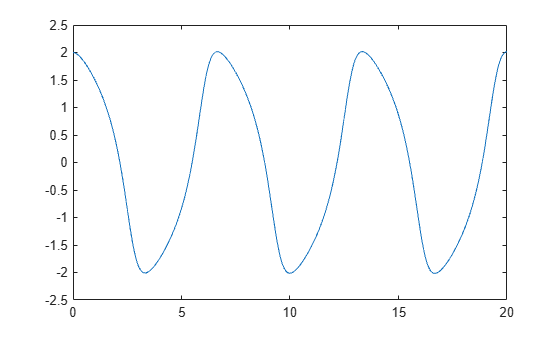# ode45

## 语法

[t,y] = ode45(odefun,tspan,y0)
[t,y] = ode45(odefun,tspan,y0,options)
[t,y,te,ye,ie] = ode45(odefun,tspan,y0,options)
sol = ode45(___)

## 说明

[t,y] = ode45(odefun,tspan,y0)（其中 tspan = [t0 tf]）求微分方程组 $y\text{'}=f\left(t,y\right)$ 从 t0 到 tf 的积分，初始条件为 y0。解数组 y 中的每一行都与列向量 t 中返回的值相对应。所有 MATLAB® ODE 求解器都可以解算 $y\text{'}=f\left(t,y\right)$ 形式的方程组，或涉及质量矩阵 $M\left(t,y\right)y\text{'}=f\left(t,y\right)$ 的问题。求解器都使用类似的语法。ode23s 求解器只能解算质量矩阵为常量的问题。ode15s 和 ode23t 可以解算具有奇异质量矩阵的问题，称为微分代数方程 (DAE)。使用 odeset 的 Mass 选项指定质量矩阵。ode45 是一个通用型 ODE 求解器，是您解算大多数问题时的首选。但是，对于刚性问题或需要较高准确性的问题，其他 ODE 求解器可能更适合。有关详细信息，请参阅选择 ODE 求解器。

[t,y] = ode45(odefun,tspan,y0,options) 还使用由 options（使用 odeset 函数创建的参数）定义的积分设置。例如，使用 AbsTol 和 RelTol 选项指定绝对误差容限和相对误差容限，或者使用 Mass 选项提供质量矩阵。
[t,y,te,ye,ie] = ode45(odefun,tspan,y0,options) 还求 (t,y) 的函数（称为事件函数）在何处为零。在输出中，te 是事件的时间，ye 是事件发生时的解，ie 是触发的事件的索引。对于每个事件函数，应指定积分是否在零点处终止以及过零方向是否重要。为此，请将 'Events' 属性设置为函数（例如 myEventFcn 或 @myEventFcn），并创建一个对应的函数：[value,isterminal,direction] = myEventFcn(t,y)。有关详细信息，请参阅 ODE 事件位置。

sol = ode45(___) 返回一个结构体，您可以将该结构体与 deval 结合使用来计算区间 [t0 tf] 中任意点位置的解。您可以使用上述语法中的任何输入参数组合。

## 示例

${y}^{\prime }=2t.$

tspan = [0 5]; y0 = 0; [t,y] = ode45(@(t,y) 2*t, tspan, y0);

plot(t,y,'-o')van der Pol 方程为二阶 ODE

${y}_{1}^{\prime \prime }-\mu \left(1-{y}_{1}^{2}\right){y}_{1}^{\prime }+{y}_{1}=0,$

$\begin{array}{cl}{y}_{1}^{\prime }& ={y}_{2}\\ {y}_{2}^{\prime }& =\mu \left(1-{y}_{1}^{2}\right){y}_{2}-{y}_{1}.\end{array}$

function dydt = vdp1(t,y) %VDP1 Evaluate the van der Pol ODEs for mu = 1 % % See also ODE113, ODE23, ODE45. % Jacek Kierzenka and Lawrence F. Shampine % Copyright 1984-2014 The MathWorks, Inc. dydt = [y(2); (1-y(1)^2)*y(2)-y(1)]; 

[t,y] = ode45(@vdp1,[0 20],[2; 0]);

plot(t,y(:,1),'-o',t,y(:,2),'-o') title('Solution of van der Pol Equation (\mu = 1) with ODE45'); xlabel('Time t'); ylabel('Solution y'); legend('y_1','y_2')ode45 仅适用于使用两个输入参数（ty）的函数。但是，通过在函数外部定义参数并在指定函数句柄时传递这些参数，可以传入额外参数。

${y}^{\prime \prime }=\frac{A}{B}ty.$

$\begin{array}{cl}{y}_{1}^{\prime }& ={y}_{2}\\ {y}_{2}^{\prime }& =\frac{A}{B}t{y}_{1}.\end{array}$

function dydt = odefcn(t,y,A,B) dydt = zeros(2,1); dydt(1) = y(2); dydt(2) = (A/B)*t.*y(1); end 

A = 1; B = 2; tspan = [0 5]; y0 = [0 0.01]; [t,y] = ode45(@(t,y) odefcn(t,y,A,B), tspan, y0);

plot(t,y(:,1),'-o',t,y(:,2),'-.')function dydt = odefcn(t,y,A,B) dydt = zeros(2,1); dydt(1) = y(2); dydt(2) = (A/B)*t.*y(1); end

yprime = @(t,y) -2*y + 2*cos(t).*sin(2*t);

y0 = -5:5;

tspan = [0 3]; [t,y] = ode45(yprime,tspan,y0);

plot(t,y) grid on xlabel('t') ylabel('y') title('Solutions of y'' = -2y + 2 cos(t) sin(2t), y(0) = -5,-4,...,4,5','interpreter','latex')• 您无法求解具有多个初始条件的方程组。该方法仅适用于求解一个具有多个初始条件的方程。

• 求解器在每步选择的时间步基于方程组中需要采取最小步长的方程。这意味着求解器可以采取小步长来满足一个初始条件的方程，但其他方程如果单独求解的话将使用不同步长。尽管如此，同时针对多个初始条件进行求解通常比使用 for 循环分别求解方程更快。ft = linspace(0,5,25); f = ft.^2 - ft - 3; gt = linspace(1,6,25); g = 3*sin(gt-0.25); 

myode 函数接受额外的输入参数以计算每个时间步的 ODE，但 ode45 只使用前两个输入参数 ty

function dydt = myode(t,y,ft,f,gt,g) f = interp1(ft,f,t); % Interpolate the data set (ft,f) at time t g = interp1(gt,g,t); % Interpolate the data set (gt,g) at time t dydt = -f.*y + g; % Evaluate ODE at time t 

tspan = [1 5]; ic = 1; opts = odeset('RelTol',1e-2,'AbsTol',1e-4); [t,y] = ode45(@(t,y) myode(t,y,ft,f,gt,g), tspan, ic, opts); 

plot(t,y)van der Pol 方程为二阶 ODE

${y}_{1}^{\prime \prime }-\mu \left(1-{y}_{1}^{2}\right){y}_{1}^{\prime }+{y}_{1}=0.$

tspan = [0 20]; y0 = [2 0]; sol = ode45(@vdp1,tspan,y0)
sol = struct with fields: solver: 'ode45' extdata: [1x1 struct] x: [0 1.0048e-04 6.0285e-04 0.0031 0.0157 0.0785 0.2844 0.5407 ... ] y: [2x60 double] stats: [1x1 struct] idata: [1x1 struct] 

x = linspace(0,20,250); y = deval(sol,x);

plot(x,y(1,:))sol_new = odextend(sol,@vdp1,35); x = linspace(20,35,350); y = deval(sol_new,x); hold on plot(x,y(1,:),'r')## 输入参数

function dydt = odefun(t,y) dydt = 5*y-3; end

$\begin{array}{l}y{\text{'}}_{1}={y}_{1}+2{y}_{2}\\ y{\text{'}}_{2}=3{y}_{1}+2{y}_{2}\end{array}$

function dydt = odefun(t,y) dydt = zeros(2,1); dydt(1) = y(1)+2*y(2); dydt(2) = 3*y(1)+2*y(2); end

• 如果 tspan 有两个元素 [t0 tf]，求解器将返回在该区间内的每个内部积分步计算的解。

• 如果 tspan 包含两个以上的元素，[t0,t1,t2,...,tf]，求解器将返回在给定点处计算的解。但是，求解器不会精确步进到 tspan 中指定的每个点。此时，求解器使用自己的内部积分步来计算解，然后在 tspan 中请求的各点处计算解。在指定点处生成的解与在每个内部积分步计算的解具有相同的准确度级别。

指定多个中间点对计算效率影响甚微，但对于大型系统可能会影响内存管理。

• 如果 tspan 包含多个中间点 [t0,t1,t2,...,tf]，则指定的点表示了问题的规模，这可能影响求解器使用的 InitialStep 的值。因此，根据您是将 tspan 指定为二元素向量还是包含中间点的向量，求解器获得的解可能有所不同。

• tspan 中的初始值和最终值用于计算最大步长 MaxStep。因此，更改 tspan 中的初始值或最终值可能导致求解器使用不同步长序列，从而可能会更改解。

options 结构体，指定为结构体数组。使用 odeset 函数创建或修改 options 结构体。有关与每个求解器兼容的选项列表，请参阅 ODE 选项摘要

## 输出参数

• 如果 tspan 包含两个元素 [t0 tf]，则 t 包含用于执行积分的内部计算点。

• 如果 tspan 包含两个以上元素，则 ttspan 相同。

sol.x

sol.y

sol.solver

sol.xe

sol.ye

sol.xe 中的事件相对应的解。

sol.ie

Events 选项中指定的函数所返回的向量的索引。这些值指示求解器检测到的事件。

## 算法

ode45 基于显式 Runge-Kutta (4,5) 公式 Dormand-Prince 对。这是一种单步求解器 – 在计算 y(tn) 时，该求解器仅需要最靠近该时间点的前一时间点处的解 y(tn-1) , 

 Dormand, J. R. and P. J. Prince, “A family of embedded Runge-Kutta formulae,” J. Comp. Appl. Math., Vol. 6, 1980, pp. 19–26.

 Shampine, L. F. and M. W. Reichelt, “The MATLAB ODE Suite,” SIAM Journal on Scientific Computing, Vol. 18, 1997, pp. 1–22.